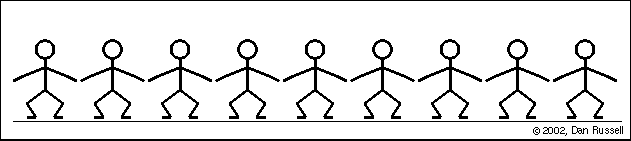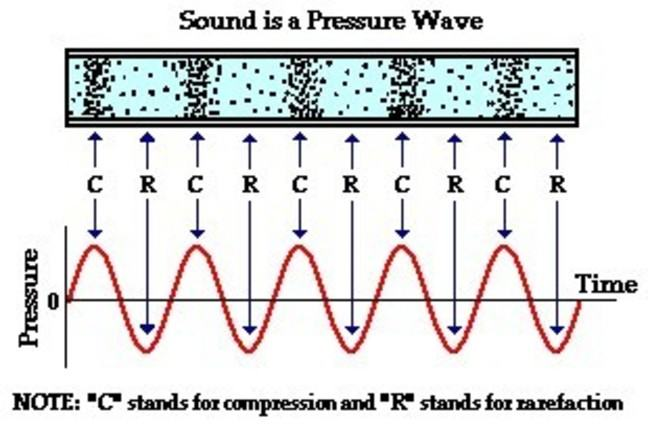## Want to keep learning?

This content is taken from the University of York's online course, Engineering the Future: Creating the Amazing. Join the course to learn more.
2.12

## University of York# How DBs work

In How Environmental Noise is Measured we discussed how noise is largely characterised by measuring the Sound Pressure Level (SPL) and reporting this in decibels (dB).

Using decibels as our measurement unit means that we can measure sound on a logarithmic scale. To understand why a logarithmic scale is useful for measuring sound we first need to think about what ‘sound’ actually is.

## What is sound?

Sound is a variation in pressure that is propagated as a wave through a medium. This can be any medium, but most of the time we think about sound as variations in air pressure, travelling through air. Of course, sound can also travel through solids, such as wood and metal, or liquids. The properties of the medium affect the speed at which sound travels; that’s why everything sounds slightly odd underwater for example.

A nice way of trying to visualise a sound wave travelling through air is to imagine that the air particles are represented by a chain of golf balls connected together by springs. If one of the golf balls is disturbed, this sets up a chain reaction - each ball in turn oscillates around its resting point (equilibrium position), and the disturbance is passed along the chain as a longitudinal wave.

It’s similar to the waves that sometimes happen in a crowd of people watching a sports event - a few people start the wave and it travels around the stadium, but the people themselves don’t move from their seating positions.When the first air particle disturbed in the wave moves towards its nearest neighbour, the air pressure at that point increases - called compression. As the air particle moves back again through its equilibrium position and to the other side, the air pressure between the two neighbouring particles decreases - this is rarefaction.As the sound wave travels, regularly alternating areas of compression and rarefaction are set up.This means that if we capture the sound wave at one stationary point (e.g. the point where you put your noise level meter) we can plot the air pressure variations against time, as illustrated in the picture above.

Image Credits: Many thanks to Dan Russell for allowing us to use his fantastic acoustics animations. Dan works at Penn State University, USA, and has produced loads of really interesting animations about sound.

## How do we measure sound amplitude?

We discovered in the previous video that measuring environmental noise involves taking an average level at one location over a fixed period of time. We need to do this by calculating the average level of the sound wave in a particular way called the root mean square (RMS).

If we just took a simple mean average, by adding up all the pressure values over time and then dividing by the number of values we had, the positive values would in effect cancel out the negative values and leave us with an average of zero. But that doesn’t make much sense for us as we are trying to capture the effective amplitude of a wave. Taking the root mean square (RMS) value produces a better measure of the amount of pressure variation in the sound wave. To do this we first square all the pressure values, which has the effect of making every value positive (so there are no longer any negatives cancelling out positives). Then we take the average of the squares, and then take the square root of that average. This gives us an average value that much better represents the amplitude of the sound wave.

Calculating the RMS pressure amplitude of a sound wave is fairly simple, but it turns out that the range of pressure levels that we might want to measure in the world can vary over a huge range because the human ear is incredibly sensitive.

The human ear can detect sound pressure levels that range between:

• Just under 10 micropascals (10 μPa or 10 × 10- 5 Pa): the threshold of human hearing

• Just over greater than 20 pascals (20 Pa): the threshold of pain

• That’s a range of over 2,000,000 (two million!) (note 1 Pascal is equal to a pressure of 1 newton per square metre (1 N m–2)) When we have a large range to measure it’s often much more efficient and clearer to use a logarithmic scale rather than a linear scale. On a linear scale the change between two values is based on the difference between them; for example moving from 20 to 30 on a linear scale represents a difference of 10.

On a logarithmic scale however, the changes between two values are based on multiplication (or orders of magnitude). On our logarithmic Decibel scale a sound measured at 30dB has a 10 times greater acoustic pressure amplitude than a sound measured at 20dB.

There are lots of different things that engineers and scientists measure on a logarithmic scale because of the large range of values they need to capture, such as:

• The strength of earthquakes

• The intensity of light

• Sound pressure levels.

Here are some common examples of approximate sound pressure levels in decibels which should give you a good idea of how sensitive, and incredible, human hearing really is.

Description dB SPL How many times more intense than the threshold of hearing
Near total silence/threshold of human hearing 0 dB -
Whisper 20 dB 100 times
“Normal” conversation at 1m 60 dB 100,000
Heavy traffic at 10m 80 dB 10,000,000
A lawnmower 90 dB 1,000,000,000
Night club peak levels 110 dB 100,000,000,000
Jet engine at 100m 120 dB 1,000,000,000,000
Gunshot at gunner’s ear 140 dB 100,000,000,000,000
Rocket launch (one mile from the launch site!) 180 dB 1,000,000,000,000,000,000

## Over to you

Can you find any more examples of the dB levels of example sounds? Post them below.

Bear in mind that any noise above approximately 80dB can cause hearing loss. The risk of hearing loss depends on the sound pressure level of the sound and the amount of time you are exposed to it. Most countries have regulations about the level and length of time that workers are allowed to be exposed to noise in order to guard against hearing damage.

Can you find out what the regulations are in your country? Share your findings below.